×
Log in to StudySoup
Get Full Access to Penn State - PHYS 214 - Document
Join StudySoup for FREE
Get Full Access to Penn State - PHYS 214 - Document

Already have an account? Login here
×
Reset your password

PENN STATE / Physics / PHYS 214 / a shopping cart is given an initial velocity

# a shopping cart is given an initial velocity Description

##### Uploaded: 05/04/2017
10 Pages 41 Views 0 Unlocks
Reviews

## What is the acceleration of the ball of yarn?## What is the ball’s displacement?## What is his average velocity along the trail?Exercise 1: 1. A biker travels at an average speed of 18 km/h along a 0.30 km straight segment of a bike  path. How  much time does the biker take to travel this segment? 2. A hiker travels south along a straight path for 1.5 h with an average speed of 0.75 km/h and  then  travels north for 2.5 h with an average speed of 0.90 km/h. WhatWe also discuss several other topics like concept development practice page 9-2
Don't forget about the age old question of Is this not how marriage is like?
If you want to learn more check out imran merchant
We also discuss several other topics like plants lose water from their aboveground surfaces in the process of transpiration
Don't forget about the age old question of chemistry: form ws7.5.2a
If you want to learn more check out a monoprotic acid in a 0.1 m solution ionises to 0.001
is the hiker’s displacement for the  total trip? 3. A stroller walks the first half of a straight 1.0 km trail at a steady pace of 0.75 m/s, east. He  walks the  second half at a constant stride of 0.60 m/s, east. What is his average velocity along the trail? 4. A shopping cart is given an initial velocity of 2.0 m/s and undergoes a constant acceleration  of 3.0  m/s2. What is the magnitude of the cart’s displacement after the first 4.0 s of its motion? 5. A skater glides off a frozen pond onto a patch of ground at a speed of 1.8 m/s. Here she is  slowed at  a constant rate of 3.00 m/s2. How fast is the skater moving when she has slid 0.37 m across  the  ground? 6. A sports car traveling at 27.8 m/s slows at a constant rate to a stop in 8.00 s. What is the  displacement  of the sports car in this time interval? 7. A toy car is given an initial velocity of 5.0 m/s and experiences a constant acceleration of 2.0 m/s2.  What is the final velocity after 6.0 s? 8. A soccer ball is moving horizontally at a speed of 3.0 m/s. It then undergoes a constant  negative  acceleration. After 4.0 s, the ball is moving at 1.5 m/s. What is the ball’s displacement? 9. A race car accelerates from 0.0 m/s to 30.0 m/s with a displacement of 45.0 m. What is the  car’s  acceleration? 10. A kitten pushes a ball of yarn rolling toward it at 1.00 cm/s with its nose, displacing the ball  of yarn  17.5 cm in the opposite direction in 2.00 s. What is the acceleration of the ball of yarn?11. A rock is thrown downward from the top of a cliff with an initial speed of 12 m/s. If the rock  hits the  ground after 2.0 s, what is the height of the cliff? (Disregard air resistance. a = −g = −9.81  m/s2.) 12. A rock is thrown straight upward with an initial velocity of 24.5 m/s where the acceleration  due to  gravity has a magnitude of 9.81 m/s2. What is the rock’s displacement after 1.00 s? 13. A coin released at rest from the top of a tower hits the ground after falling 1.5 s. What is the speed of  the coin as it hits the ground? (Disregard air resistance. a = −g = −9.81 m/s2.) 14. A rock is thrown straight upward with an initial velocity of 9.6 m/s in a location where the  acceleration  due to gravity has a magnitude of 9.81 m/s2. To what height does it rise? 15. Someone throws a rubber ball vertically upward from the roof of a building 8.00 m in height. The ball  rises, then falls. It just misses the edge of the roof, and strikes the ground. If the ball is in the  air for  3.00 s, what was its initial velocity? (Disregard air resistance. a = −g = −9.81 m/s2.) Exercise 2: 1) An astronaut of mass 90 kg moves at a velocity of 6ms-1 and bumps into a stationary  astronaut mass 100kg. Howfast do the two astronaut move together after the collision? 2. A trolley of mass 3 kg moving at a velocity of 2ms-1 collides with another trolley of mass 0.5kg which is moving ata velocity of 1ms-1 in the same direction. If the 0.5 kg trolley moves at a velocity of 2.5ms-1 in the same direction afterthe collision, what is the velocity of the 3kg trolley? 3. Block A of mass 5 kg is moving with velocity 2 m s-1 and collides with another stationery block B of unknown mass.  After the collision, block A moves with velocity 0.5 m s-1. Given that the collision is elastic. Find  the Momentum of block B after the collision.4. A truck travels at a velocity of 15 m s-1 collides head-on with a car that travels at 30 m s-1.  The mass of the truckand the car are 6000 kg and 1500 kg respectively. What is the final  velocity of the two vehicles after the collision if they stick together? 5. Car A of mass 100 kg traveling at 30 m s-1  collides with Car B of mass 90 kg traveling at 20 m s-1 in front of it.Car A and B move separately after collision. If Car A is still moving at 25 m s-1 after collision, determine the velocityof Car B after collision. 6. An arrow of mass 150 g is shot into a wooden block of mass 450 g lying at rest on a smooth  surface. At themoment of impact, the arrow is travelling horizontally at 15 ms-1. Calculate the  common velocity after the impact7. An instructor fires a pistol which has a massof 1.5 kg. If the bullet weighs 10 g and itreaches a velocity of 300 m s-1 after shooting, what is the recoil  velocity of thepistol? Essay Question.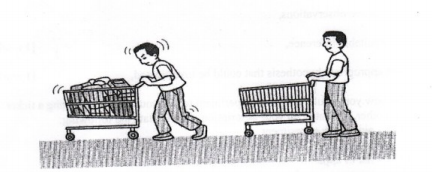In a supermarket, firdaus help his mother to  push an empty trolley to carry the things she  intends to buy. After shopping, he pushes the  trolley which is full to the cashier’s counter.  Firdaus noticed that it is more difficult to push full trolley compared to an empty one.Based on the observation, a) make one suitable inference, [1 mark] b) state one appropriate hypothesis that could be investigate [1 mark] c) describe how you would design an experiment to test your hypothesis using an inertia  balance, G-clamp and other apparatus. In your description, clearly state the following :  i) aim of the experiment  ii) variables in the experiment  iii) list of apparatus and materials  iv) arrangement of the apparatus  v) the procedures of the experiment, which includes the method of controlling the  manipulated variable and the method of measuring the responding variable.  vi) the way you would tabulate the data  vii) the way you would analyse the data [10 marks] Answer :  Option 1: Using an inertia balance Inference : Inertia depends on mass Hypothesis: The larger the mass, the bigger the inertia When the mass increases, the inertia increases Aim of the experiment: To study the effect of mass on the inertia of an  object Variables: Manipulated: Mass, mResponding: Period of oscillation, T (inertia) Constant: Stiffness of the inertia balance Apparatus/Materials:  Inertia balance, masses for the inertia balance, G clamp, stopwatch. Arrangement: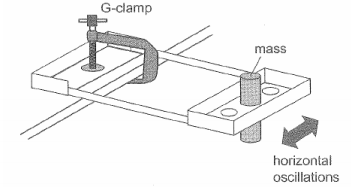Procedure: 1. The inertia balance is set up. 2. One piece of mass is placed. The inertia balance  is oscillated in horizontal plane. 3. The time for 10 complete oscillations is measured using a stopwatch. 4. Steps 2 and 3 are repeated using two and three  masses on the inertia balance. 5. A graph of T2 versus number of masses, n is  drawn. Results:Graph of T2 versus m: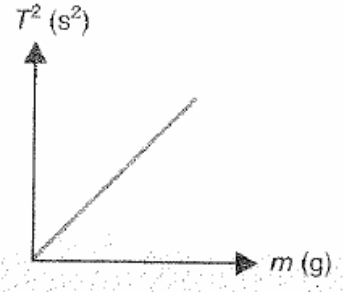Discussion:  The graph of T2 versus m shows a straight line  passing through the origin.   The period of oscillation increases (directly  porpotional) with the mass of the load;   an object with a large mass has a large inertia. Conclusion:  Objects with a large mass have a large inertia.   This is the reason why it is difficult to set an object of large mass in motion or to stop it.   The hypothesis is valid.Option 2: Using a jigsaw blade Apparatus/Materials:  Jigsaw blade, G-clamp, stopwatch, and  plasticine spheres of mass 20 g, 40 g, 60 g, 80 g,  and 100 g Arrangement: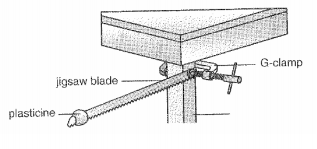Procedure: 1. One end of jigsaw blade clamped to leg of table  with G-clamp. 2. A 20 g plasticine ball is fixed at one end of blade. 3. The blade is displaced horizontally and released  to oscillate. The time for 10 complete oscillations  measured with stopwatch. This step repeated.. 4. Steps 2 and 3 are repeated with 40 g, 60 g, 80 g,  and 100 g. 5. A graph of T2 versus mass of load, m is drawn. Result: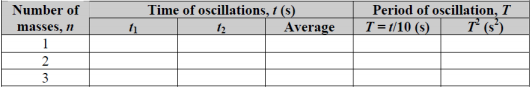Graph of T2 versus m: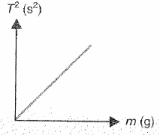Discussion: The graph of T2 versus m shows a straight line passing through the origin. This means that the  period of oscillation increases with the mass of the load; that is, an object with a large mass has a large inertia. Conclusion: Objects with a large mass have a large inertia. This is the reason why it is difficult to set an  object of large mass in motion or to stop it. The hypothesis is valid.

Page ExpiredIt looks like your free minutes have expired! Lucky for you we have all the content you need, just sign up here